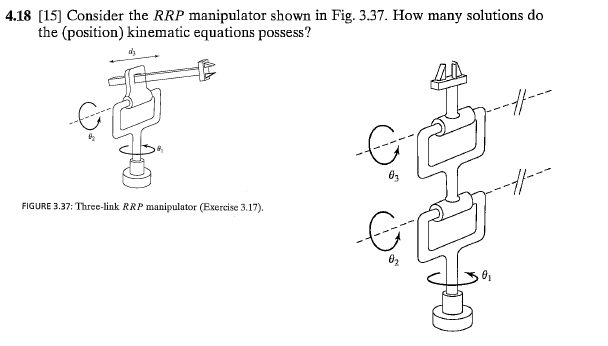# How many solutions are there to this?

I'm studying for an exam and I came across these questions where you have to determine the number of possible inverse kinematic equations like(qs from Craig Introduction To Robotics, 3rd Edition)

How exactly do you find the number of solutions? I know how to find the DH parameters and then the inverse kinematics but is there some way to calculate the number of solutions quickly like this question suggests? Like, I can visualize it (4 for the second one) but is there a proper way?